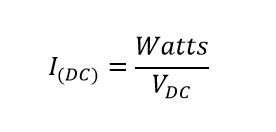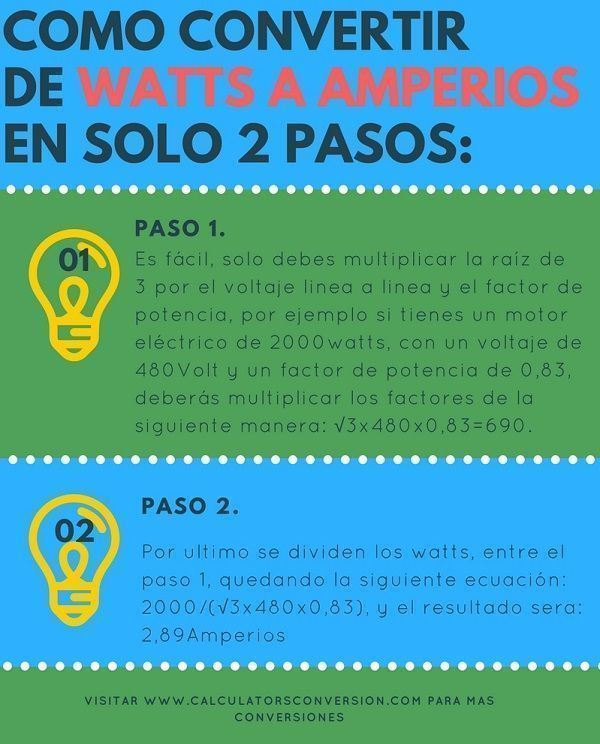# Watts to Amps- Calculator, conversion, examples, table and formula

With this tool you can convert from Watts to Amps , you will also find the formula and the explanation of how to perform the conversion.

Some  examples , the most common power factors , the main definitions and finally the  table of equivalences from Watts to Amps .

## ⚡Formulas to convert from Watts to Amps AC and DC

With the following formulas you can convert Watts to Amps manually , if you want to convert automatically you can use the  initial Watts to Amps calculator .

### Watts to amps (DC) formula:Where :

• Watts = This is the real power of electrical equipment  and is commonly associated with resistive (DC) and inductive (AC) systems is the useful power and most equipment is designated with this power, this unit alone does not mean much Because it requires time to have a real meaning, having time converts kWh into a measure of the energy consumed by the equipment, despite the above you will almost always find the Watts and not the kWh on the nameplate of any electrical equipment

The value of the Watts is specific to each team and the larger the value, the greater the energy consumption it will have.

• L-N = Line-to-neutral voltage is a characteristic of electrical systems and it is very important to be attentive to it every time it is used in the formula for single-phase or two-phase AC systems. The AC voltage is the one that is commonly used in the equipment of a home or industry to power the final equipment.

If you have the line-to-line voltage and want to convert it to neutral-line you can use the conversion  tool  L-N  to  L-L

• L-L = Line-to-line voltage, is commonly used in three-phase AC systems and is a data that you can find on each unit’s characteristic plates , this data is only required when using the three-phase Watts Amps formula.

If you only have the single-phase voltage you can convert it to three-phase using this conversion  tool  L-L  to  L-N

• DC = This voltage only applies to DC (Direct Current Systems) systems and once you have it you just have to enter it in the formula for DC equipment.

Generally the devices that have this type of voltage are LED luminaires , DC motors, electronics such as cell phones, televisions, computers etc, these devices always have to convert from AC / DC through a transformer, this in order to be able to connect them to AC voltage, That is common in homes.

• AC1Ø = This is the value you want to find in case the load is monophasic .
• AC2Ø = Value to be found when the load is Bifasica.
• AC3Ø = Value to be found for three-phase systems.
• FP = The power factor is the very important term in the electricity sector, that is, generation, transmission, distribution and use, as long as working with AC (alternating current), this value is what differentiates the direct current DC from AC alternating current and denotes how efficient a device is to consume energy , in other words it shows how much real energy watts a device consumes.

Now, the closer the power factor is to the unit, the greater the active power P it consumes. Therefore, the power factor affects the output power of the device.

## ☝How to calculate or convert Watts to Amps in electrical systems

Before converting from watts to amps you should be clear about the formula of watts to amps, in this article we show what are the steps to perform the conversion.

To convert from watts to amps, you should know three variables : The watts, the type of voltage (AC or DC), the number of phases for AC electrical systems (Three-phase, single-phase or two-phase) and the power factor.

Commonly the watts and the voltage appear on the electrical equipment’s characteristic plates (See figure 1), however explicitly the number of phases and the power factor do not appear, so these latter values ​​must often be deduced in the case of number of phases or estimate based on the typical power factor tables  .

For the previous case we can deduce that the number of phases of the equipment is 2 (two-phase) , this is deduced by the type of connection and the voltage (See figure 2), in fact knowing the number of phases in this way depends a lot on the experience ( Voltages in the world )

On the other hand there is the power factor which is typical of each equipment and is on the nameplate, in case you do not have this factor you can see the tables of typical power actors .

Conversions can be done manually using the watts to amps formula  or automatically using the watts to amps calculator. The steps for calculating watts to amps are as follows:

## ⭐Steps to calculate or convert from Watts to Three Phase Amps:### Step 1:

You must identify which is the formula that serves you (AC, DC, three-phase, single-phase, two-phase), then it is only to replace each variable, in the case of three-phase systems, you must only multiply the root of 3 by the line-to-line voltage and the power factor , for example if you have a 2000watts electric motor, with a voltage of 480Volt and a power factor of 0.83, you must multiply the factors as follows: √3x480x0.83 = 690.

### Step 2:

Finally, the watts are divided between step 1, leaving the following equation: 2000 / (√3x480x0.83), and the result will be: 2.89 amps.

Note: if you do not know the power factor you can have the typical values ​​for many devices  here

## 🔥Examples of Watts at amps:

To help you understand the conversion of Watts to amps we present many examples and exercises of watts to amps.

### Example 1, convert the watts of a wifi antenna to amps:

In a summer house there is a Wi-Fi antenna with 800Watts (AC), the antenna is connected to a neutral line voltage of 127V and according to the equipment’s rating plate they have a power factor of 0.98 , how much amperage will the Wi-Fi antenna have? .

Rta: //  The easiest way is to perform the calculation automatically with the watts to amps conversion tool.

The other form of conversion is to perform the calculation manually, the first thing you should do is identify the watt-amp formula  that works best for you, in this case it will be the formula for single-phase AC systems, then you must enter the variables as they appear in the formula, in summary only the single-phase voltage must be multiplied by the power factor (127Vx0.98) , then the watts must be divided by the result of the previous equation as follows: 800 / (127 × 0.98) = 6.43A.

### Example 2, how to convert watts to from a forklift to amps:

In a warehouse there is a three-phase forklift (AC), which has a consumption of 5000 Watts , with a line voltage of 240V and a power factor of 0.82 , what amperage will the forklift have ?.

Rta: //  It’s simple, you just have to multiply the voltage, by the root of 3 and by the power factor (√3x240x0.82) , which will result in 340.9, then you must divide the watts by the result, of as follows: 5000 / 340.9 = 14.7 amps . This result was reached by taking the watts formula at three-phase amps.

### Example 3, conversion from watts to amps for a metal halide lamp:

A winery has a metal halide lamp that has a power of 400Watts bifasica (AC) , a line-to-line voltage of 208V and a neutral line voltage of 120V , with a power factor of 0.93 , what amperage does the lamp have? .

Rta: //  To get the result you must divide the watts between the multiplication of the neutral line voltage, the power factor and the number 2, as follows: 400 / (2x120x0.93) , which will result in: 1 , 79A.

## 🥇 Table watts to amps conversion, equivalence, transformation

These are the tables of watts to amps for most common equipment , in them you will see that the conversion of watts to amps is different due to variables such as voltage, power factor and even internal functioning of the equipment.

### Table of watts to amps for appliances:

In this table you can see the equivalent of watts to amps for the most used appliances in a home.

 Portable home appliance (Appliances) Watts Amps Laptop 65 – 100 <0.5 Mobile phone charger <12 <0.5 Teapot 3000 13 Satellite television box 30 <0.5 Printer fifty <0.5 Radio 40 <0.5 Radiator 2000 8.5 DVD Player 28 <0.5 Hair dryer 2200 10.0 Cordless phone charger 10 <0.5 Computer monitor 100 <0.5 Desktop 700 3.0 Television 42 “HD 120 0.5 Game console <200 0.86 Washing machine 2200 10 Toaster 2000 9.0 Dryer 2500 11.0 Dishwasher 2200 10.0 Iron 2800 12.5 Microwave 1000 4.5 Vacuum cleaner 2000 9.0

### Table of watts at amps for 120V:

In this table you can see the equivalent of watts to amps for a voltage of 120V and a power factor of 0.8.

 Watt power Ampere Current 75 0.78 125 1.30 175 1.82 225 2.34 275 2.86 325 3.39 375 3.91 425 4.43 475 4.95 525 5.47 625 6.51 725 7.55 825 8.59 925 9.64 1025 10.68 1125 11.72 1225 12.76 1325 13.80 1425 14.84 1525 15.89 1625 16.93 1725 17.97

### Table of watts to amps in 12V:

In this table you can find the conversion in DC for 12V equipment.

 Watts Amps fifteen 1.25 twenty 1.67 25 2.08 30 2.50 35 2.92 40 3.33 Four. Five 3.75 fifty 4.17 55 4.58 55 4.58 65 5.42 75 6.25 85 7.08 95 7.92 105 8.75 115 9.58 125 10.42 135 11.25 145 12.08 155 12.92 165 13.75 175 14.58

## 👌Most common conversions:

### The equivalence of 1 watts in amps:

There are several answers for this conversion depending on the type of AC or DC current, power factor etc, however the simplest answer is  0.01 Amps, for AC voltage, power factor equal to 1 and 120V voltage.

### How many amps are 1000 Watts:

The simplest answer will be 8.33 Amp with an AC voltage, a power factor of 1 and a voltage of 120V.

### 1500 Watts how many amps are:

Like the previous 1500Watts conversion are 12.5 Amps, with an AC voltage, a fp of 1, a single phase voltage of 120V.

### 600 Watts to how many amps is equivalent:

They are 5 single phase AC amps, with a voltage of 120 V and a power factor of 1.

### 2000 Watts to how many amps is equivalent:

The equivalence is 16.67 single phase amps of 120Volt AC, a power factor of 1.

## 🎯Definitions of watts, amps and volts:

### Amps:

Amps are the flow of electricity that is measured as electric current . You can see the amps as the flow of water through a pipe. The more water passes through the pipe, the stronger the current.

### Volts:

Volts are used to determine how much force is needed to make electric current flow . According to the previous example, you could think of volts as the pressure imposed on the water in a pipe, which causes the force for the water to flow.

### Watts (watts):

The amps multiplied by volts are the watts, which is the measure used to determine the amount of energy consumed by electrical equipment. The higher the wattage, the greater the power in watts. In terms of the example of water, watts referred to the amount of water that has been released.

## 💡How to use the calculator:

Initially you must enter the Watts that you want to convert, this information can be found on the nameplate of each equipment, then choose the type of system and the number of phases from several options ( single – phase -1F-AC, single- phase three-phase-2L -AC, biphasic -2F-AC, trifasico -3F-AC or DC), the difference between trifilar-2L-2F monophasic and biphasic system is that the source voltage is different , for the case of the source 2L is monophasic , while for 2F the source is three-phase, the above although these systems could have the same voltage.

Then you must choose the power factor , this value is specific to each device, however here we show some values ​​that could be useful , after choosing the power factor you must select the voltage , the voltages are divided into LL (Line-Line) , LN (Line-Neutral) or DC Voltage , you must choose the voltage to which you will connect the equipment,  finally click on calculate to finish or restart to enter new values.